# Algebra II : Simplifying Expressions

## Example Questions

← Previous 1 3 4 5 6 7 8

### Example Question #1 : Simplifying Expressions

Simplify x(4 – x) – x(3 – x).

Possible Answers:

3x

1

0

x

x2

Correct answer:

x

Explanation:

You must multiply out the first set of parenthesis (distribute) and you get 4x – x2. Then multiply out the second set and you get –3x + x2. Combine like terms and you get x.

x(4 – x) – x(3 – x)

4x – x2 – x(3 – x)

4x – x2 – (3x – x2)

4x – x2 – 3x + x2 = x

### Example Question #1 : How To Divide Trinomials

Divide: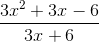Possible Answers: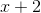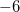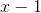Correct answer:Explanation:

Factor the numerator and denominator: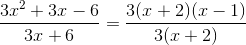Cancel the factors that appear in both the numerator and the denominator: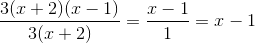### Example Question #1 : Simplifying Expressions

Simplify: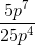Possible Answers: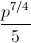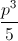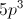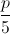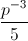Correct answer:Explanation: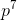and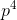cancel out, leaving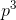in the numerator. 5 and 25 cancel out, leaving 5 in the denominator

### Example Question #1 : Simplifying Expressions

Simplify the following: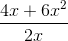Possible Answers: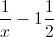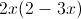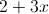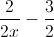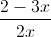Correct answer:Explanation:First, let us factor the numerator: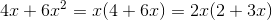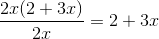### Example Question #8 : Monomials

Find the product: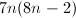Possible Answers: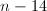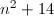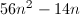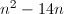Correct answer:Explanation:

First, mulitply the mononomial by the first term of the polynomial: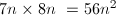Second, multiply the monomial by the second term of the polynomial: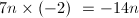Add the terms together: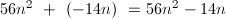### Example Question #4 : Simplifying Expressions

Multiply, expressing the product in simplest form: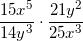Possible Answers: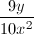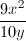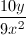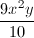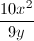Correct answer:Explanation:

Cross-cancel the coefficients by dividing both 15 and 25 by 5, and both 14 and 21 by 7: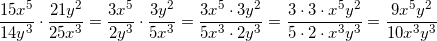Now use the quotient rule on the variables by subtracting exponents: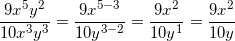### Example Question #5 : Simplifying Expressions

Simplify the following: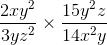Possible Answers: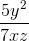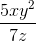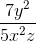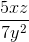Correct answer:Explanation:

In this problem, you have two fractions being multiplied. You can first simplify the coefficients in the numerators and denominators. You can divide and cancel the 2 and 14 each by 2, and the 3 and 15 each by 3: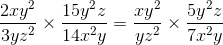You can multiply the two numerators and two denominators, keeping in mind that when multiplying like variables with exponents, you simplify by adding the exponents together: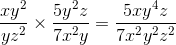Any variables that are both in the numerator and denominator can be simplified by subtracting the numerator's exponent by the denominator's exponent. If you end up with a negative exponent in the numerator, you can move the variable to the denominator to keep the exponent positive: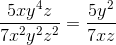### Example Question #3 : How To Factor A Variable

Factor the expression: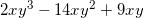Possible Answers: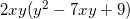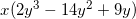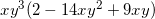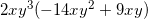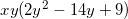Correct answer:Explanation:

To find the greatest common factor, we need to break each term into its prime factors: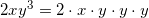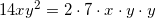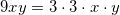Looking at which terms all three expressions have in common; thus, the GCF is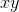. We then factor this out:### Example Question #9 : Monomials

Expand: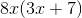Possible Answers: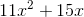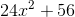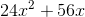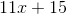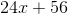Correct answer:Explanation:

To expand, multiply 8x by both terms in the expression (3x + 7).

8x multiplied by 3x is 24x².

8x multiplied by 7 is 56x.

Therefore, 8x(3x + 7) = 24x² + 56x.

### Example Question #1 : Binomials

Simplify: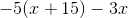Possible Answers: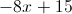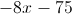None of the other answers are correct.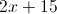Correct answer:Explanation:

First, distribute –5 through the parentheses by multiplying both terms by –5.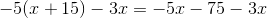Then, combine the like-termed variables (–5x and –3x).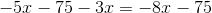← Previous 1 3 4 5 6 7 8

### All Algebra II Resources